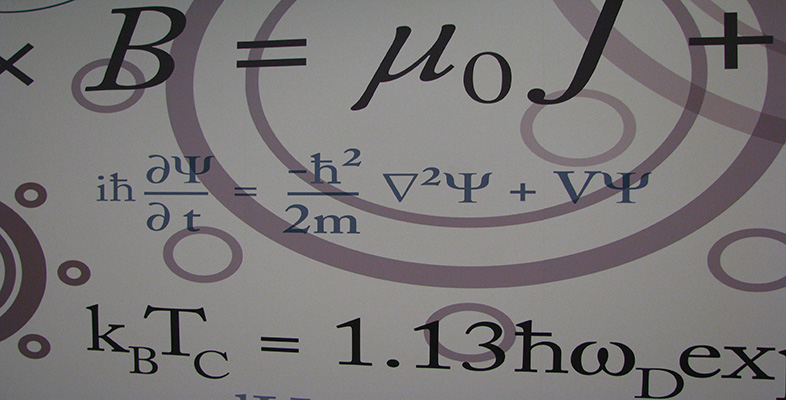Number systems

This free course is available to start right now. Review the full course description and key learning outcomes and create an account and enrol if you want a free statement of participation.

Free course

2.3 Complex arithmetic

Arithmetical operations on complex numbers are carried out as for real numbers, except that we replace i2 by −1 wherever it occurs.

Example 1

Let z1 = 1 + 2i and z2 = 3 − 4i. Determine the following complex numbers.

• (a)  z1 + z2

• (b)  z1z2

• (c)  z1z2

• (d)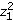Solution

Using the usual rules of arithmetic, with the additional property that i2 = −1, we obtain the following.

• (a)  z1 + z2 = (1 + 2i) + (3 − 4i) = (1 + 3) + (2 − 4)i = 4 − 2i

• (b)  z1 − z2 = (1 + 2i) − (3 − 4i) = (1 − 3) + (2 + 4)i = − 2 + 6i

• (c)  z1z2 = (1 + 2i)(3 − 4i) = 3 + 6i − 4i − 8i2 = 3 + 2i + 8 = 11+ 2i

• (d)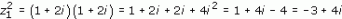Exercise 8

Determine the following complex numbers.

• (a)  (3 − 5i) + (2 + 4i)

• (b)  (2 − 3i)(−3 + 2i)

• (c)  (5 + 3i)2

• (d)  (1 + i)(7 + 2i)(4 − i)

Solution

• (a)  (3 − 5i) + (2 + 4i) = 5 − i

• (b)  (2 − 3i)(−3 + 2i) = −6 + 9i + 4i − 6i2 = 13i

• (c)

•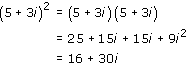• (d)  We find

•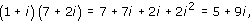• so

•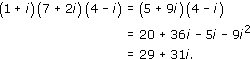Example 1 illustrates how we add, subtract and multiply two given complex numbers. We can apply the same methods to two general complex numbers z﻿1 = x1 + iy1 and z2 = x2 + iy2, and obtain the following formal definitions of addition, subtraction and multiplication in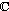.

Definitions

Let z1 = x1 + iy1 and z2 = x2 + iy2 be any complex numbers. Then the following operations can be applied.

addition    z1 + z2 = (x1 + x2) + i(y1 + y2)

subtraction    z1z2 = (x1x2) + i(y1y2)

multiplication    z1z2 = (x1x2y1y2) + i(x1y2 + y1x2)

With these definitions, most of the usual rules of algebra still hold, as do many of the familiar algebraic identities. For example,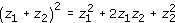and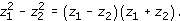There is no need to remember or look up these formulas. For calculations, the methods of Example 1 may be used.

An obvious omission from this list of definitions is division. We return to division after discussing the complex conjugate and modulus of a complex number.

M208_6